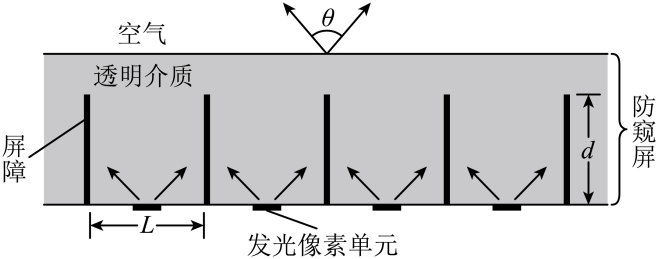(1) 若把发光像素单元视为点光源, 要求可视角度 $\theta$ 控制为 $60^{\circ}$, 求屏障的高度 $d$;
(2) 若屏障高度 $d=1.0 \mathrm{~mm}$, 且发光像素单元的宽度不能忽略, 求像素单元宽度 $x$ 最小为多少时, 其可视 角度 $\theta$ 刚好被扩为 $180^{\circ}$ (只要看到像素单元的任意一点, 即视为能看到该像素单元)。